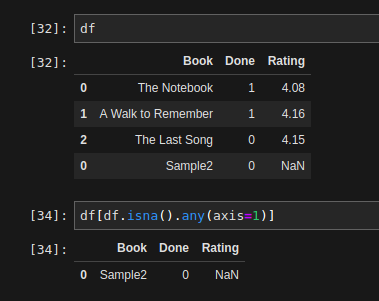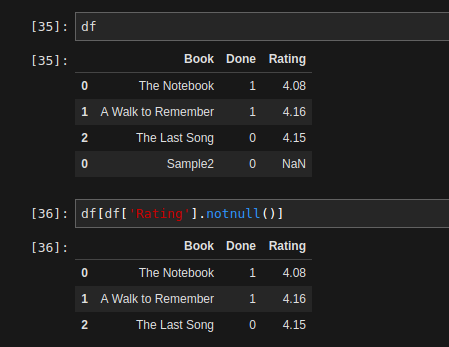Get rows with NaNs in any column

`df[df.isna().any(axis=1)]`Get dataframe excluding all NaNs in a particular Column

`df[df['<col_name>'].notnull()]`Get counts of number of Nulls per column

`df.isnull().sum()`

Increase width of columns displayed in Jupyter Notebooks

• This is possibly one of my most used commands for every notebook that involves dataframes.

`pd.set_option('display.max_colwidth',400)`